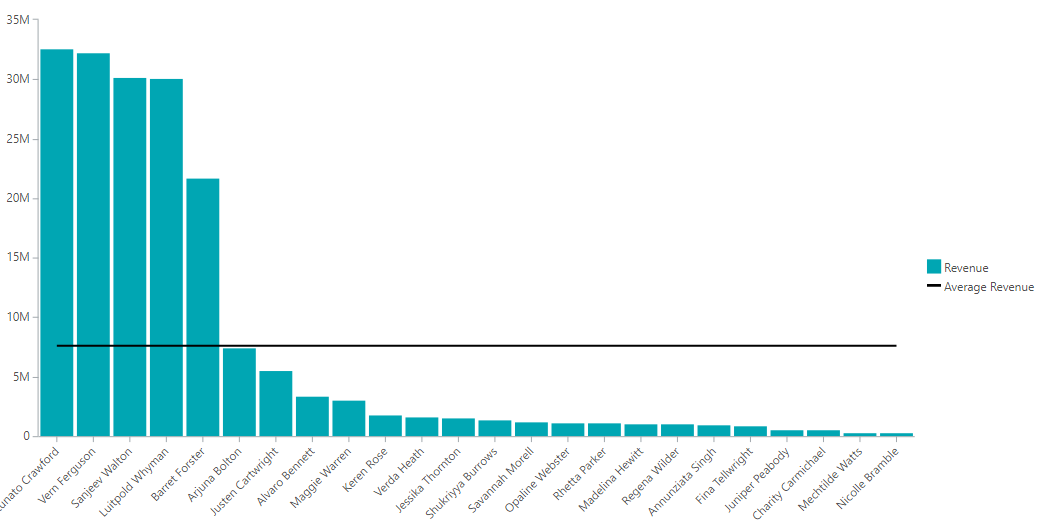# December 17th: Smart Calculations (1) - Total and Average

When you pick the calculations tab in the TARGIT Client, your first option is to use one of the built-in smart calculations.

Here is the full list of Smart calculations: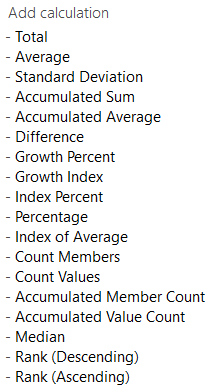In this article, we will focus on the first 3 - Total, Average, and Standard Deviation.

### Total (just the visible data)

In this table, it might seem strange to click the total - since it will just be a duplicate of the total already shown in the table.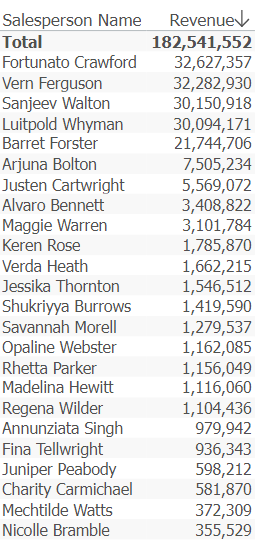However, if you click the total and then add a visibility agent that hides everyone below 1.000.000, you will see the difference: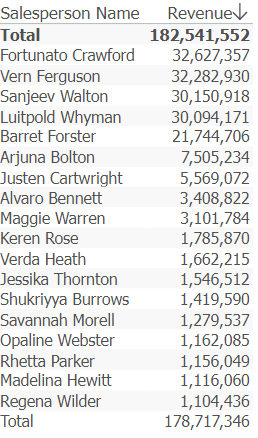As you can see, the totals are not the same now.

That's because the smart calculation Total includes the (v) modifier by default, which means you get the total of the visible data.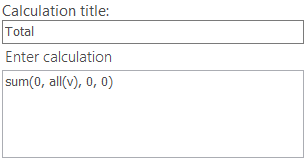So use the Total when you've used the visibility agent!

### Average (row or column)

If you add the average to the same table (which will turn up as a row like the total), you will notice that the syntax of the average holds the same (v) modifier:That means you can do an average based on the visible data (in case you have hidden some data with the visibility agent).

Notice also that you have two options when you look at the calculation: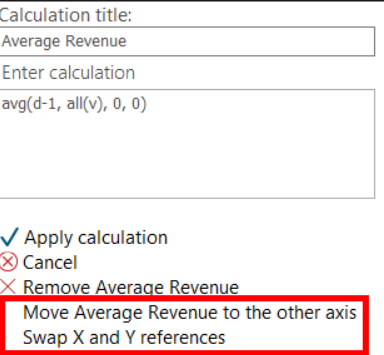If you click both - you transform the calculated row holding the average to a calculated column like this: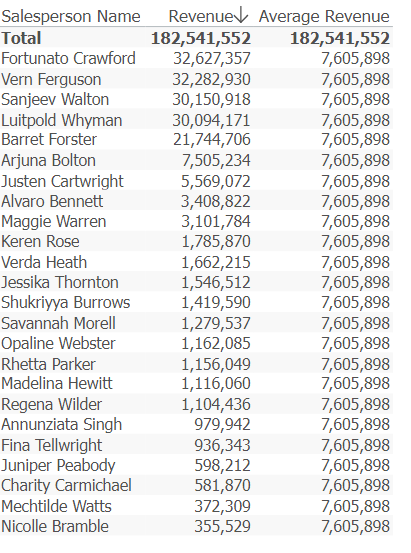So now you can make a graph like this: